# Exponents

Exponent review:  an or BaseExponent

 Exponential notation Example
 Base     Exponent an= a ∙ a ∙ a ∙ a … a 24 = 2 ∙ 2 ∙ 2 ∙ 2  = 16 Read “a to the nth” or “the nth power of a.” Read “2 to the 4th.”

Properties of exponents:

 Name Rule Example
 Product rule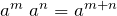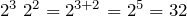Quotient rule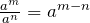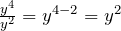Power rule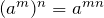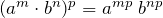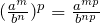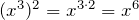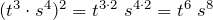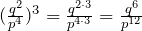Negative exponent a-n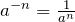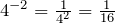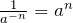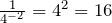Zero exponent   a0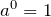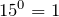One exponent   a1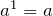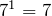,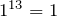Fractional exponent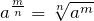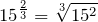• Product rule: when multiplying two powers with the same base, keep the base and add the exponents.

am an = am + n            an   or   BaseExponent

 Example: 23 22 = (2 · 2 · 2) (2 · 2) = 25 = 32 Or 23 22 = 23 + 2 = 25 = 32 A short cut, am an = am + n

• Quotient rule: when dividing two powers with the same base, keep the base and subtract the exponents.Example: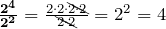Or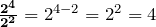A short cut,This law can also show that why a0 = 1 (zero exponent a0):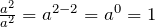• Power rule: when raising an expression to a power, we multiply each exponent inside the parentheses by the power outside the parentheses.

(am)n = amn,        (am · bn)p = amp bnp,Example: (43)2 = (43) (43) = (4 · 4 · 4) (4 · 4 · 4) = 46 = 4096 Or (43)2 = 43 ∙ 2 = 46 = 4096 A short cut, (am)n = amn
 Example: (2 · 3)2 = (2 · 3) (2 · 3) = 6 ∙ 6 = 36 Or (2 · 3)2 = 22 32 = 4 ∙ 9 = 36 A short cut , (a · b)n = an bn
 Example: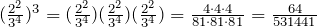Or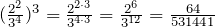A short cut,• Negative exponent: a negative exponent is the reciprocal of the number with a positive exponent.a−n is the reciprocal of an.

 Example: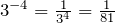Example: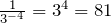• Fractional exponent: a fractional exponent is a different way of writing a radical (i.e. root) sign. The base is first taken to the exponent of m, then the nth root is found to obtain the power.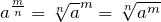Example: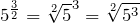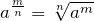Example 1.5.1

Simplify (do not leave negative exponents in the answer).

 1)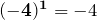2)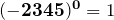3)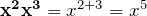4)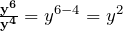5)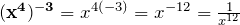,6)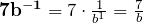,7)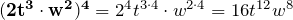8)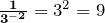9)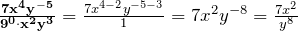,,10)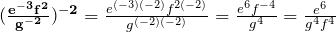,# Simplifying Exponential Expressions

• Remove parentheses using “power rule” if necessary.             (am bn)p = amp bnp
• Regroup coefficients and variables.
• Use “product rule” and “quotient rule”.                                   am an = am + n ,• Simplify.
• Use the “negative exponent” rule to make all exponents positive if necessary.

Example 1.5.2

Simplify.

 1)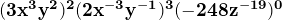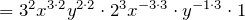Remove brackets.,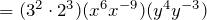Regroup coefficients and variables.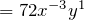Simplify.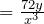Make exponent positive.,2)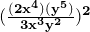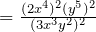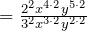Remove brackets.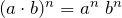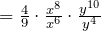Regroup coefficients and variables.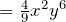Simplify.Example 1.5.3

Evaluate for   a = 2,   b = 1,   c = -1.

 1)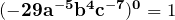2)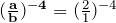Substitute 2 for a and 1 for b,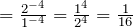,,3)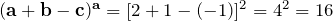Substitute 2 for a and 1 for b, and -1 for c.

# Scientific Notation

Scientific notation is a special format to concisely express very large and small numbers.

Example:

300,000,000 = 3 × 108 m/sec. The speed of light.

0.00000000000000000016 = 1.6 × 10-19 C. An electron.

Scientific notation: a product of a number between 1 and 10 and a power of 10.

 Scientific notation Example
 N × 10±n 1 ≤ N < 10 67504.3 = 6.75043 × 104 n – integer Standard form Scientific notation

Writing a number in scientific notation:

 Step Example
 Move the decimal point after the first nonzero digit. 0.0079                  37213000 Determine n (the power of 10) by counting the number of places you moved the decimal. n = 3                     n = 7 If the decimal point is moved to the right: × 10–n 0.0079 = 7.9 × 10-3 3 places to the right. If the decimal point is moved to the left: × 10n 37213000. = 3.7213 × 107 7 places to the left.

Example 1.5.4

Write in scientific notation.

1)     2340000 = 2340000. = 2.34× 106

6 places to the left, × 10n

2)     0.000000439 = 4.39 × 10-7

7 places to the right, × 10-n

Example 1.5.5

Write in standard (or ordinary) form.

1)     6.4275 × 104 = 64275

2)     2.9 × 10-3 = 0.0029

# Practice questions

1. Evaluate:

a. 4x2 + 5y,    for x = 1,    y = 4

b. (2a)3 – 3b,    for a = 5,    b = 6

2. Simplify (do not leave negative exponents in the answer):

a. (-92)1

b. y4 y3

c.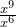d. 13a-1

e. (3a2 · b3)4

f.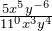g.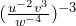3. Write in scientific notation:

a. 45,600,000

b. 0.00000523

4. Write in standard (or ordinary) form:

a. 3.578 × 103

b. 4.3 × 10-5